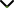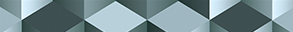# Fixed Point Theory and its Applications in Ordinary and Fractional Differential and Integral Equations

Publishing date
01 May 2023
Status
Closed
06 Jan 2023

Guest Editors

1Atilim University, Ankara, Turkey

3National Hsinchu University of Education, Hsinchu, Taiwan

4Sivas Cumhuriyet University, Sivas, Turkey

5Niš University, Niš, Serbia

This issue is now closed for submissions.

# Fixed Point Theory and its Applications in Ordinary and Fractional Differential and Integral Equations

This issue is now closed for submissions.

## DescriptionIt has been 100 years since Banach published the first metric fixed point theorem. Today, metric fixed point theory is one of the hottest research topics not only in applied mathematics but also in nonlinear functional analysis and topology. In qualitative sciences, almost all questions can be transferred into the metric fixed point problem f(x)=x for some suitable function f. The main goal of the fixed point theory is to understand the existence of a fixed point first. After guaranteeing the existence of a fixed point, we look for its uniqueness. For instance, the existence and uniqueness of certain differential equations, under suitable conditions can be reduced to the existence and uniqueness of a fixed point. This is why the metric fixed point theory has been so densely studied in qualitative sciences, in particular, computer science, engineering, physics, etc.

Over the last decades, fixed point theory has been applied to differential and integral equations successfully. Keeping in mind that almost all real-world problems can be transformed into the problem of differential and integral equations, we can conclude the importance of the metric fixed point theory in qualitative science and technology. More precisely, differential/difference/integral equations appear in many scientific issues to comprehend the distinct phenomena in the economy, engineering, chemical technology, finance, domain theory, optimal control, and so on. Besides all these, fixed point theory has been used in fractional differential equations as well as fractional integral equations. Based on these discussions and observations, we underline the fact that metric fixed point theory is one of the most useful and effective mathematical analysis tools to construct the existence and uniqueness of various problems modeled by nonlinear relations. Hence, the existence and uniqueness problems of (fractional) differential/difference/integral equations are studied using fixed point theory. Metric fixed points have begun to take shape and sharply develop in different senses. With the invention of several new contraction mapping, various new abstract spaces have been discovered and studied. In connection to the advances in metric fixed point theory, several new results (dealt with fixed point theory) in many disciplines have been recorded.

This Special Issue aims to collect theoretical developments on fixed metric point theory and its applications in fractional and ordinary differential/difference/integral equations. We welcome original research and review articles.

Potential topics include but are not limited to the following:

• Fixed point theory and its applications
• Topological fixed-point theory
• Metric fixed point theory
• The fixed points of certain operators on various abstract spaces
• Applications in difference equations
• Applications in integral equations
• Applications in ordinary/partial differential equations
• Applications in fractional differential equations
• Nonlinear problems with fixed point theory approaches
• Best proximity point and applications
• Controllability problems for nonlinear systemsJournal metrics
See full report
Acceptance rate32%
Submission to final decision58 days
Acceptance to publication25 days
CiteScore2.400
Journal Citation Indicator1.150
Impact Factor1.281Author guidelinesEditorial boardDatabases and indexing

Article of the Year Award: Outstanding research contributions of 2021, as selected by our Chief Editors. Read the winning articles.